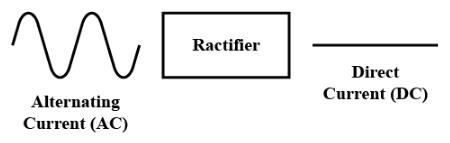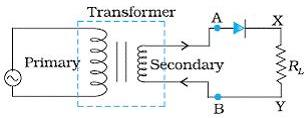Courses

# Test: Applications Of PN Diode

## 10 Questions MCQ Test Physics For JEE | Test: Applications Of PN Diode

Description
This mock test of Test: Applications Of PN Diode for JEE helps you for every JEE entrance exam. This contains 10 Multiple Choice Questions for JEE Test: Applications Of PN Diode (mcq) to study with solutions a complete question bank. The solved questions answers in this Test: Applications Of PN Diode quiz give you a good mix of easy questions and tough questions. JEE students definitely take this Test: Applications Of PN Diode exercise for a better result in the exam. You can find other Test: Applications Of PN Diode extra questions, long questions & short questions for JEE on EduRev as well by searching above.
QUESTION: 1

### The knee voltage of a-n junction diode is 0.8 V and the with of the depletion layer is 2 μm. What is the electric field in the depletion layer?​

Solution:

Knee voltage- it is that forward voltage beyond which current start increasing rapidly, but below knee voltage variation of forward current and applied voltage is linear.
The electric field in a region is given by, E=V/l.
where V is the potential and l is the length or distance of the region in which it has to be measured.
now, E=V/l
E=0.8/2×10-6 m
E= 0.4 MV/m

QUESTION: 2

### An electronic device which converts a.c power into d.c power is

Solution:

A rectifier is a device which converts a.c (alternating current) to d.c( direct current), which flows in only one direction. This process is known as Rectification.
This is done using a p-n junction diode.
A p-n junction diode allows electric current in only forward bias condition and blocks electric current in reverse bias conditions.
In other words, a diode allows electric current in one direction. This unique property of diode allows it to act like a Rectifier.QUESTION: 3

### In a half wave rectifier, the secondary coil S of the transformer is connected to​

Solution:In a half wave rectifier, the secondary coil S of the transformer is connected to​ both junction diode and a load resistance.

QUESTION: 4

A 50 Hz a.c. is rectified with full wave rectifier, what is the output frequency?​

Solution:

In full wave rectification the input frequency is doubled. Because all the negative components in the AC input signal are converted into positive components. Hence, the positive components are doubled.
Therefore in full wave rectification output frequency will be 100 Hz i.e. double that of input frequency 50 Hz.

QUESTION: 5

The conductivity of a photosensitive semiconductor

Solution:

Photoconductivity, as a well-known optical and electrical phenomenon in semiconductor, is an effect that the electrical conductivity increases due to the absorption of light radiation.

QUESTION: 6

Zener diodes are used as

Solution:

Zener Diodes can be used to produce a stabilised voltage output with low ripple under varying load current conditions. By passing a small current through the diode from a voltage source, via a suitable current limiting resistor (RS), the zener diode will conduct sufficient current to maintain a voltage drop of Vout.

QUESTION: 7

The current that exists in the circuit of a photodiode even when no visible light is made incident on it is called

Solution:

In physics and in electronic engineering, dark current is the relatively small electric current that flows through photosensitive devices such as a photomultiplier tube, photodiode, or charge-coupled device even when no photons are entering the device; it consists of the charges generated in the detector when no outside radiation is entering the detector. It is referred to as reverse bias leakage current in non-optical devices and is present in all diodes. Physically, dark current is due to the random generation of electrons and holes within the depletion region of the device.

QUESTION: 8

The efficiency of a full wave rectifier is

Solution:

Efficiency of half-wave and full wave rectifier is given by
ηh​=40.6% and ηf​=81.2%
So,  ηhf​​​=40.6/81.2 ​=1/2​
∴f/n ​=2ηh​
Hence, the efficiency of a full wave rectifier is double of half wave-rectifier.

QUESTION: 9

The energy of radiation emitted by LED is

Solution:

This energy is emitted in the form of heat and light. The electrons dissipate energy in the form of heat for silicon and germanium diodes but in gallium arsenide phosphide (GaAsP) and gallium phosphide (GaP) semiconductors, the electrons dissipate energy by emitting photons.

QUESTION: 10

A diode as a rectifier converts:​

Solution:

A diode as a rectifier is used for converting AC to DC.
Following are the applications of the diode as a rectifier:
It is used for the mixing of signals.
It is used for the detection of signals.
Used in lighting systems.
When a diode is used as a rectifier, it acts as a two-lead semiconductor which allows current to pass from only one direction.